# Dispersion of Light

## Dispersion of Light

the dependence of the index of refraction n of a material on the frequency v (or the wavelength λ) of light or the dependence of the phase velocity of the light waves on frequency. A consequence of the dispersion of light is the resolution into a spectrum of a beam of white light passing through a prism. The study of this spectrum led I. Newton to the discovery of the dispersion of light (1672). For a material that is transparent in a given region of the spectrum, n increases as v increases (as λ decreases), to which the distribution of colors in a spectrum corresponds. Such a ratio of n to λ is cajled normal dispersion of light.

The way in which n varies with the wavelength becomes much more complex near absorption bands. For example, in a thin prism made of cyanine dye (see Figure 1), in the absorption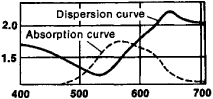Figure 1. Dependence of the indexes of refraction (solid line) and absorption (dotted line) on wavelength (in mμ) for a thin prism made of cyanine dye

region the red rays are refracted more strongly than the violet rays; the least refracted color is green, followed by blue (so-called anomalous dispersion of light). Each substance has its own absorption bands, and the general behavior of the index of refraction is determined by the distribution of the absorption bands in the spectrum.

The refraction of light in a substance arises because of a change in the phase velocity of light; the index of refraction n = c0/c, where c0 is the velocity of light in a vacuum and c is its phase velocity in a given medium. According to the electromagnetic theory of light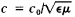, where ε is the dielectric constant and μ, is the magnetic permeability. In the optical region of the spectrum μ, is very close to 1 for all substances. Therefore,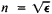, and the dispersion of light can be explained by the dependence of ε on frequency. This dependence is associated with the interaction of the electromagnetic field of the light wave with atoms and molecules, giving rise to absorption; in this case the index of refraction becomes the complex value ñ = n + ik, where k characterizes absorption. Electron oscillations are of the greatest significance in the visible and ultraviolet regions of the spectrum; ion oscillations are of the greatest significance in the infrared region.

According to classical concepts, the electric field of a light wave acts on the electrons of atoms or molecules; as a result, the electrons perform forced oscillations at a frequency equal to the frequency of the incident wave. As the frequency of the light wave approaches the natural oscillation frequency of the electrons, the phenomenon of resonance arises, bringing about the dependence of ε on frequency, as well as the absorption of light. This theory explains well the relationship between dispersion of light and the absorption bands. To obtain quantitative agreement with experimental values, in classical theory it was necessary to introduce an empirical constant (the “oscillator force”) for each absorption line. According to the electron theory, the following approximation formulas are valid: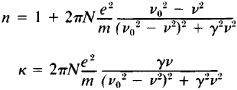where N is the number of particles per unit volume, e and m are the charge and mass of an electron, and γ is the attenuation factor. The dependence of n and κ on v/v0 is shown in Figure 2.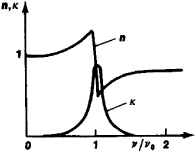Figure 2. Graph of the dependence of n and κ on v/v0

Quantum theory has confirmed the qualitative results of classical theory and made possible the connection of the constants with other characteristics of the electron shell of an atom (their wave functions in various energy states). It also explained some peculiarities of the dispersion of light that are observed in cases where a significant number of atoms exists in an excited state (so-called negative dispersion of light).

The dispersion of light in transparent materials, which is used in optical instruments, is of a great importance in the design of spectral instruments to produce satisfactory spectra and of achromatic lenses and prisms, as well as in eliminating chromatic aberration.

Rotary dispersion is the change in the angle of rotation φ of the plane of polarization, depending on the wavelength λ. In transparent substances the angle φ usually increases as λ decreases. For some mediums an approximation of the relationship is given by Biot’s law: φ = K2 (K is the constant for a given substance). Rotary dispersion of this type is called normal. In the region of light absorption the behavior of rotary light dispersion is much more complex. In this case the angle φ can attain enormously large values (anomalous rotary dispersion).

### REFERENCES

Landsberg, G. S. Optika, 4th ed. Moscow, 1957. (Obshchii kurs fiziki, vol. 3.)
Gorelik, G. S. Kolebaniia i volny, 2nd ed. Moscow-Leningrad, 1959.

M. D. GALANIN

References in periodicals archive ?
The hardness of diamond and its high dispersion of light - giving the diamond its characteristic fire - make it useful for industrial applications and desirable as jeweller y.
The cut has three attributes; brilliance (the total light reflected by a diamond), fire (the dispersion of light into the colours of the spectrum) and finally scintillation (the flashes of light or sparkle when the diamond is moved).
In electromagnetic theory, the diffraction and dispersion of light are symmetric in spacetime.
To prevent this, Marcel Tolkowsky, grandfather of the current CEO, set out to define the ideal proportion of a diamond's cut to maximise brilliance and dispersion of light.
The near-Lambertian properties of Spectralon and Spectraflect deliver a uniform dispersion of light that integrates light and reduces hotspots better than other materials in coated cavity applications.
Given the radiant transportation of energy in stars, an additional factor is expected in relation to Thomson dispersion of light in free electrons, and provides the same energy radiated in the wide range of physical conditions from dwarfs to super-giants.
The number, named for Ernst Abbe, a 19th -century professor at the University of Jena and a partner in the Carl Zeiss optical glass firm, expresses an in verse ratio for the dispersion of light passing through a material.
The vision of reality which emerges from each individual ego is like the dispersion of light from a crystal chandelier," wrote Kovel in his first book, White Racism.
In our super prism the dispersion of light was twice the magnitude of a standard Newton prism," ABC Science quoted Min Gu, Director of Swinburne's Centre for Micro-Photonics, as saying.
We discuss a recently predicted mechanism whereby energy is produced by the background space non-holonomic field (the global space rotation) in Thomson dispersion of light in free electrons.

Site: Follow: Share:
Open / Close